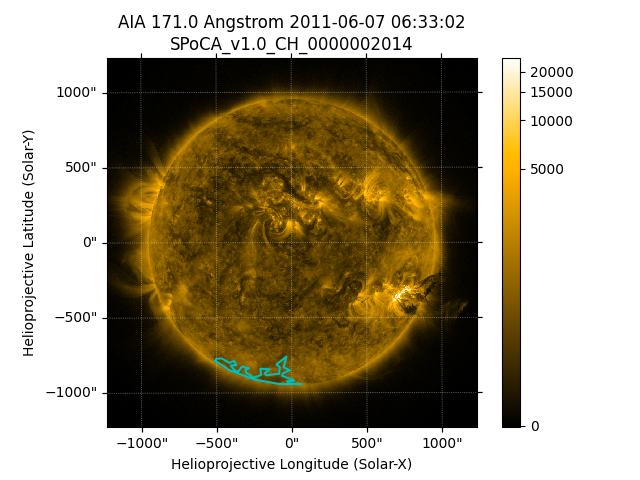# Overplotting HEK feature/event polygons on a map¶

How to overplot HEK outlines on a map.

```import matplotlib.pyplot as plt
import numpy as np

import astropy.units as u
from astropy.coordinates import SkyCoord
from astropy.time import TimeDelta

import sunpy.data.sample
import sunpy.map
from sunpy.coordinates import frames
from sunpy.net import attrs as a
from sunpy.net import hek
from sunpy.physics.differential_rotation import solar_rotate_coordinate
from sunpy.time import parse_time
```

```aia_map = sunpy.map.Map(sunpy.data.sample.AIA_171_IMAGE)
```

Look for coronal holes detected using the SPoCA feature recognition method:

```hek_client = hek.HEKClient()
start_time = aia_map.date - TimeDelta(2*u.hour)
end_time = aia_map.date + TimeDelta(2*u.hour)
responses = hek_client.search(a.Time(start_time, end_time),
a.hek.CH, a.hek.FRM.Name == 'SPoCA')
```

Let’s find the biggest coronal hole within 80 degrees north/south of the equator:

```area = 0.0
for i, response in enumerate(responses):
if response['area_atdiskcenter'] > area and np.abs(response['hgc_y']) < 80.0:
area = response['area_atdiskcenter']
response_index = i
```

Next let’s get the boundary of the coronal hole.

```ch = responses[response_index]
p1 = ch["hpc_boundcc"][9:-2]
p2 = p1.split(',')
p3 = [v.split(" ") for v in p2]
ch_date = parse_time(ch['event_starttime'])
```

The coronal hole was detected at different time than the AIA image was taken so we need to rotate it to the map observation time.

```ch_boundary = SkyCoord(
[(float(v), float(v)) * u.arcsec for v in p3],
obstime=ch_date, observer="earth",
frame=frames.Helioprojective)
rotated_ch_boundary = solar_rotate_coordinate(ch_boundary, time=aia_map.date)
```

Now let’s plot the rotated coronal hole boundary on the AIA map, and fill it with hatching.

```fig = plt.figure()